# 房价预测-机器学习的一般过程

1.划定问题

2.选择性能指标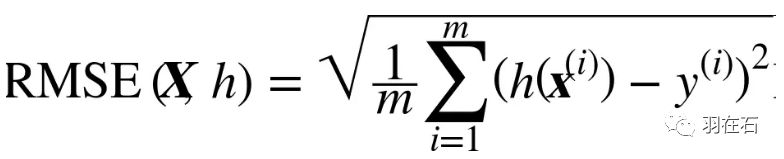1.创建工作环境

2.下载数据

import os

import tarfile

from six.moves import urllib

HOUSING_PATH = "datasets/housing"

def fetch_housing_data(housing_url=HOUSING_URL, housing_path=HOUSING_PATH):

if not os.path.isdir(housing_path):

os.makedirs(housing_path)

tgz_path = os.path.join(housing_path, "housing.tgz")

urllib.request.urlretrieve(housing_url, tgz_path)

housing_tgz = tarfile.open(tgz_path)

housing_tgz.extractall(path=housing_path)

housing_tgz.close()

fetch_housing_data()

import pandas as pd

csv_path = os.path.join(housing_path, "housing.csv")

3.查看数据结构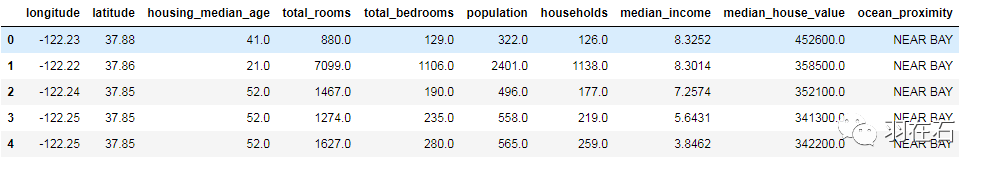info()方法可以快速查看数据的描述，包括总行数、每个属性的类型和非空值的数量。

housing.info()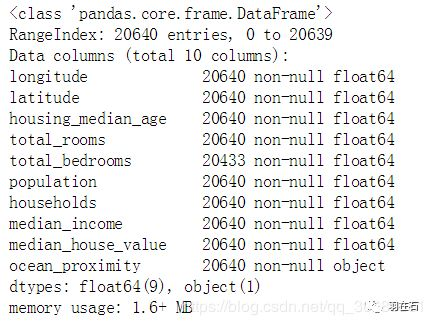housing["ocean_proximity"].value_counts()

describe()方法展示了数值属性的概括:

housing.describe()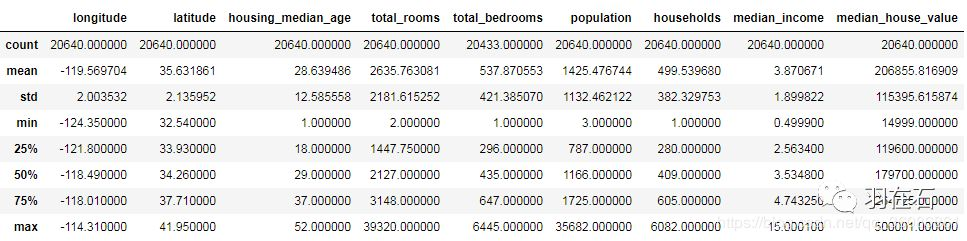count、mean、min和max几行的意思很明了。注意，空值被忽略了（所以，卧室总数是20433而不是20640）。std是标准差（揭示数值的分散度）。25%、50%、75%展示了对应的分位数：每个分位数指明小于这个值，且指定分组的百分比。例如，25%的分区的房屋年龄中位数小于18，而50%的小于29，75%的小于37。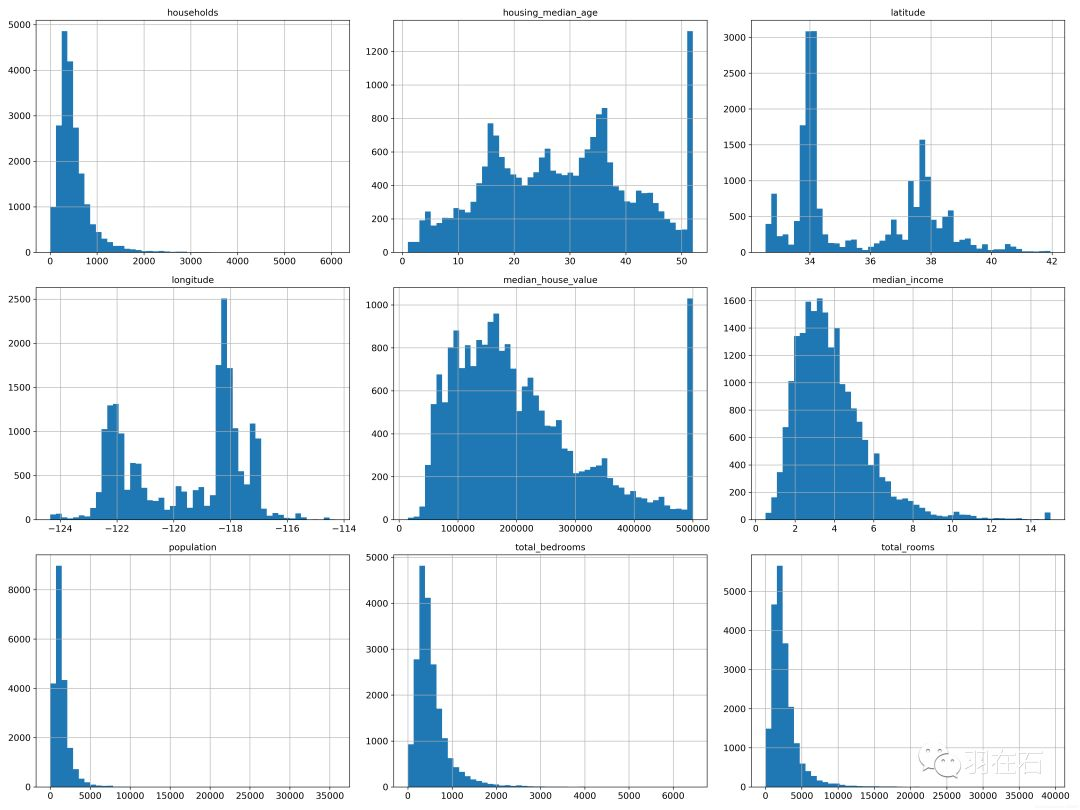4.创建测试集

(1)自己编写代码，设置随机数生成器的种子（比如np.random.seed(42)），以产生总是相同的混合指数（shuffled indices）

# to make this notebook's output identical at every run

np.random.seed(42)

import numpy as np

def split_train_test(data, test_ratio):

shuffled_indices = np.random.permutation(len(data))

test_set_size = int(len(data) * test_ratio)

test_indices = shuffled_indices[:test_set_size]

train_indices = shuffled_indices[test_set_size:]

return data.iloc[train_indices], data.iloc[test_indices]

train_set, test_set = split_train_test(housing, 0.2)

print(len(train_set), "train +", len(test_set), "test")

(2)使用hash

import hashlib

def test_set_check(identifier, test_ratio, hash):

return hash(np.int64(identifier)).digest()[-1] < 256 * test_ratio

def split_train_test_by_id(data, test_ratio, id_column, hash=hashlib.md5):

ids = data[id_column]

in_test_set = ids.apply(lambda id_: test_set_check(id_, test_ratio, hash))

return data.loc[~in_test_set], data.loc[in_test_set]

housing_with_id = housing.reset_index()   # adds an `index` column

train_set, test_set = split_train_test_by_id(housing_with_id, 0.2, "index")

housing_with_id["id"] = housing["longitude"] * 1000 + housing["latitude"]

train_set, test_set = split_train_test_by_id(housing_with_id, 0.2, "id")

(3)sklearn实现

from sklearn.model_selection import train_test_split

train_set, test_set = train_test_split(housing, test_size=0.2, random_state=42)

housing["income_cat"] = np.ceil(housing["median_income"] / 1.5)

housing["income_cat"].where(housing["income_cat"] < 5, 5.0, inplace=True)

from sklearn.model_selection import StratifiedShuffleSplit

split = StratifiedShuffleSplit(n_splits=1, test_size=0.2, random_state=42)

for train_index, test_index in split.split(housing, housing["income_cat"]):

strat_train_set = housing.loc[train_index]

strat_test_set = housing.loc[test_index]

strat_test_set["income_cat"].value_counts() / len(strat_test_set)

housing["income_cat"].value_counts() / len(housing)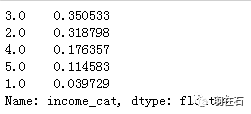def income_cat_proportions(data):

return data["income_cat"].value_counts() / len(data)

train_set, test_set = train_test_split(housing, test_size=0.2, random_state=42)

compare_props = pd.DataFrame({

"Overall": income_cat_proportions(housing),

"Stratified": income_cat_proportions(strat_test_set),

"Random": income_cat_proportions(test_set),

}).sort_index()

compare_props["Rand. %error"] = 100 * compare_props["Random"] / compare_props["Overall"] - 100

compare_props["Strat. %error"] = 100 * compare_props["Stratified"] / compare_props["Overall"] - 100

compare_props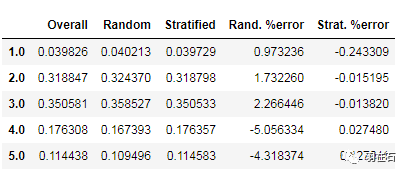for set_ in (strat_train_set, strat_test_set):

set_.drop("income_cat", axis=1, inplace=True)

housing = strat_train_set.copy()

1.地理数据可视化

housing.plot(kind="scatter", x="longitude", y="latitude", alpha=0.1)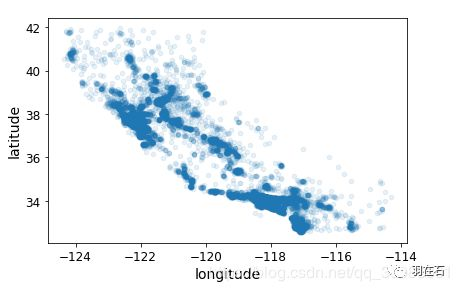housing.plot(kind="scatter", x="longitude", y="latitude", alpha=0.4,

s=housing["population"]/100, label="population",

c="median_house_value", cmap=plt.get_cmap("jet"), colorbar=True,

)

plt.legend()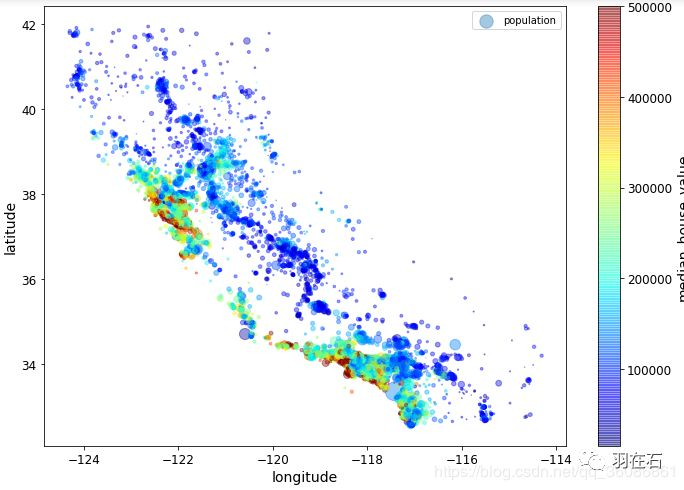2.查找关联

(1)因为数据集并不是非常大，你可以很容易地使用corr()方法计算出每对属性间的标准相关系数（也称作皮尔逊相关系数）：

corr_matrix = housing.corr()

corr_matrix["median_house_value"].sort_values(ascending=False)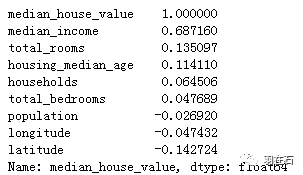(2)另一种检测属性间相关系数的方法是使用Pandas的scatter_matrix函数，它能画出每个数值属性对每个其它数值属性的图。因为现在共有11个数值属性，你可以得到112=121张图，在一页上画不下，所以只关注几个和房价中位数最有可能相关的属性。

from pandas.tools.plotting import scatter_matrix

attributes = ["median_house_value", "median_income", "total_rooms",

"housing_median_age"]

scatter_matrix(housing[attributes], figsize=(12, 8))

housing.plot(kind="scatter", x="median_income", y="median_house_value",

alpha=0.1)

plt.axis([0, 16, 0, 550000])

save_fig("income_vs_house_value_scatterplot")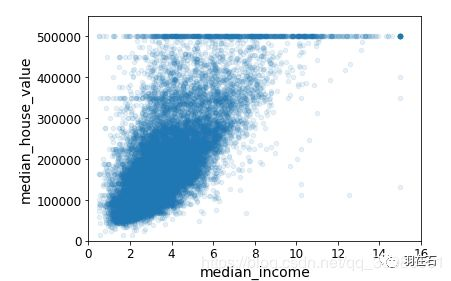3.属性组合试验

housing["rooms_per_household"] = housing["total_rooms"]/housing["households"]

housing["bedrooms_per_room"] = housing["total_bedrooms"]/housing["total_rooms"]

housing["population_per_household"]=housing["population"]/housing["households"]

corr_matrix = housing.corr()

corr_matrix["median_house_value"].sort_values(ascending=False)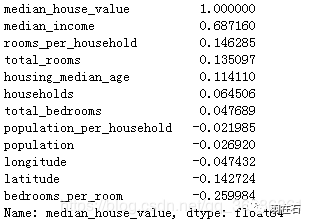housing = strat_train_set.drop("median_house_value", axis=1) # drop labels for training set

housing_labels = strat_train_set["median_house_value"].copy()

1.数据清洗

housing.dropna(subset=["total_bedrooms"])    # 选项1

housing.drop("total_bedrooms", axis=1)       # 选项2

median = housing["total_bedrooms"].median()

housing["total_bedrooms"].fillna(median)     # 选项3

Scikit-Learn提供了一个方便的类来处理缺失值：Imputer。下面是其使用方法：首先，需要创建一个Imputer实例，指定用该属性的中位数替换它的每个缺失值：

from sklearn.preprocessing import Imputer

imputer = Imputer(strategy="median")

housing_num = housing.drop("ocean_proximity", axis=1)

imputer.fit(housing_num)

imputer计算出了每个属性的中位数，并将结果保存在了实例变量statistics_中。只有属性total_bedrooms有缺失值，但是我们要确保一旦系统运行起来，新的数据中没有缺失值，所以安全的做法是将imputer应用到每个数值。使用这个“训练过的”imputer来对训练集进行转换，通过将缺失值替换为中位数：

X = imputer.transform(housing_num)

housing_tr = pd.DataFrame(X, columns=housing_num.columns)

2.处理文本和分类属性

Scikit-Learn为这个任务提供了一个转换量LabelEncoder：

housing_cat = housing[['ocean_proximity']]

from sklearn.preprocessing import OrdinalEncoder

ordinal_encoder = OrdinalEncoder()

housing_cat_encoded = ordinal_encoder.fit_transform(housing_cat)

print(encoder.classes_)

#['<1H OCEAN' 'INLAND' 'ISLAND' 'NEAR BAY' 'NEAR OCEAN']

cat_encoder = OneHotEncoder(sparse=False)

housing_cat_1hot = cat_encoder.fit_transform(housing_cat)

housing_cat_1hot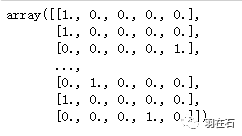3.自定义转换量

from sklearn.base import BaseEstimator, TransformerMixin

rooms_ix, bedrooms_ix, population_ix, household_ix = 3, 4, 5, 6

def __init__(self, add_bedrooms_per_room = True): # no *args or **kargs

def fit(self, X, y=None):

return self  # nothing else to do

def transform(self, X, y=None):

rooms_per_household = X[:, rooms_ix] / X[:, household_ix]

population_per_household = X[:, population_ix] / X[:, household_ix]

bedrooms_per_room = X[:, bedrooms_ix] / X[:, rooms_ix]

return np.c_[X, rooms_per_household, population_per_household,

bedrooms_per_room]

else:

return np.c_[X, rooms_per_household, population_per_household]

4.特征缩放

5.转换Pipeline

from sklearn.pipeline import FeatureUnion

num_attribs = list(housing_num)

cat_attribs = ["ocean_proximity"]

num_pipeline = Pipeline([

('selector', DataFrameSelector(num_attribs)),

('imputer', Imputer(strategy="median")),

('std_scaler', StandardScaler()),

])

cat_pipeline = Pipeline([

('selector', DataFrameSelector(cat_attribs)),

('label_binarizer', LabelBinarizer()),

])

full_pipeline = FeatureUnion(transformer_list=[

("num_pipeline", num_pipeline),

("cat_pipeline", cat_pipeline),

])

housing_prepared = full_pipeline.fit_transform(housing)

housing_prepared.shape  #(16513, 17)

1.在训练集上训练和评估

(1)线性回归模型

from sklearn.linear_model import LinearRegression

lin_reg = LinearRegression()

lin_reg.fit(housing_prepared, housing_labels)

from sklearn.metrics import mean_squared_error

housing_predictions = lin_reg.predict(housing_prepared)

lin_mse = mean_squared_error(housing_labels, housing_predictions)

lin_rmse = np.sqrt(lin_mse)

lin_rmse  #68628.19819848922

(2)决策树

from sklearn.tree import DecisionTreeRegressor

tree_reg = DecisionTreeRegressor(random_state=42)

tree_reg.fit(housing_prepared, housing_labels)

housing_predictions = tree_reg.predict(housing_prepared)

tree_mse = mean_squared_error(housing_labels, housing_predictions)

tree_rmse = np.sqrt(tree_mse)

tree_rmse   #0.0

2.使用交叉验证做更佳的评估

from sklearn.model_selection import cross_val_score

scores = cross_val_score(tree_reg, housing_prepared, housing_labels,

scoring="neg_mean_squared_error", cv=10)

tree_rmse_scores = np.sqrt(-scores)

def display_scores(scores):

print("Scores:", scores)

print("Mean:", scores.mean())

print("Standard deviation:", scores.std())

display_scores(tree_rmse_scores)

lin_scores = cross_val_score(lin_reg, housing_prepared, housing_labels,

scoring="neg_mean_squared_error", cv=10)

lin_rmse_scores = np.sqrt(-lin_scores)

display_scores(lin_rmse_scores)3.尝试新方法：随机森林

from sklearn.ensemble import RandomForestRegressor

forest_reg = RandomForestRegressor(n_estimators=10, random_state=42)

forest_reg.fit(housing_prepared, housing_labels)

from sklearn.model_selection import cross_val_score

forest_scores = cross_val_score(forest_reg, housing_prepared, housing_labels,

scoring="neg_mean_squared_error", cv=10)

forest_rmse_scores = np.sqrt(-forest_scores)

display_scores(forest_rmse_scores)1.网格搜索

from sklearn.model_selection import GridSearchCV

param_grid = [

{'n_estimators': [3, 10, 30], 'max_features': [2, 4, 6, 8]},

{'bootstrap': [False], 'n_estimators': [3, 10], 'max_features': [2, 3, 4]},

]

forest_reg = RandomForestRegressor()

grid_search = GridSearchCV(forest_reg, param_grid, cv=5,

scoring='neg_mean_squared_error')

grid_search.fit(housing_prepared, housing_labels)

param_grid告诉Scikit-Learn首先评估所有的列在第一个dict中的n_estimators和max_features的3 × 4 = 12种组合（不用担心这些超参数的含义，会在第7章中解释）。然后尝试第二个dict中超参数的2 × 3 = 6种组合，这次会将超参数bootstrap设为False而不是True（后者是该超参数的默认值）。总之，网格搜索会探索12 + 6 = 18种RandomForestRegressor的超参数组合，会训练每个模型五次（因为用的是五折交叉验证）。换句话说，训练总共有18 × 5 = 90轮！折将要花费大量时间，完成后，你就能获得参数的最佳组合。

grid_search.best_params_

grid_search.best_estimator_

cvres = grid_search.cv_results_

for mean_score, params in zip(cvres["mean_test_score"], cvres["params"]):

print(np.sqrt(-mean_score), params)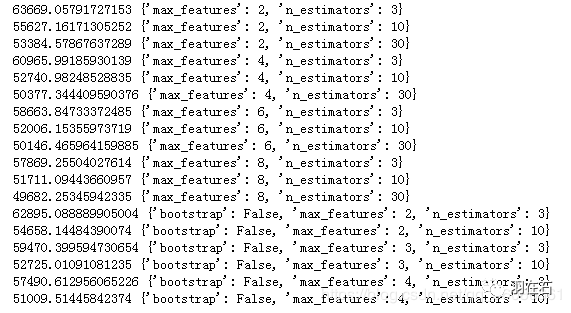2.随机搜索

3.集成方法

4.分析最佳模型和它们的误差

feature_importances = grid_search.best_estimator_.feature_importances_

extra_attribs = ["rooms_per_hhold", "pop_per_hhold", "bedrooms_per_room"]

#cat_encoder = cat_pipeline.named_steps["cat_encoder"] # old solution

cat_encoder = full_pipeline.named_transformers_["cat"]

cat_one_hot_attribs = list(cat_encoder.categories_)

attributes = num_attribs + extra_attribs + cat_one_hot_attribs

sorted(zip(feature_importances, attributes), reverse=True)

final_model = grid_search.best_estimator_

X_test = strat_test_set.drop("median_house_value", axis=1)

y_test = strat_test_set["median_house_value"].copy()

X_test_prepared = full_pipeline.transform(X_test)

final_predictions = final_model.predict(X_test_prepared)

final_mse = mean_squared_error(y_test, final_predictions)

final_rmse = np.sqrt(final_mse)   #47730.22690385927